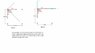# Coordinate axis (which one is correct)?

• Miike012

#### Miike012

In the problem the book the book chose to use the axis in the left diagram... but why can you not use the coordinate axis from the right diagram?

#### Attachments

•Untitled.jpg
10.5 KB · Views: 384

Well, to me it seems that the particles are pulling each other.
Because the all the particles have the same mass the first diagram is more convenient.

Here is why:
Lets label the particles.
The one on the upper right corner is A, the one on the bottom left corner is B and the one on the right bottom corner is C. The particle that is being pulled is X. Got it?

So, now that you have set up the axis the way it is shown on the first diagram do you will notice something really helpful. Particle A and particle B pull your particle X in such a way that both pull it with the same amount of force and cancel each other out! =)

Why is that? Well, if you pay close attention both A and B are at the same distance from X, but one is on the positive-Y side of the axis, and the other one on the negative side of the Y axis. If you calculate the Y-Force that A does on X, and the force that B does on X, you get that they are equal in magnitude but opposite in direction, therefore they cancel each other out.

Now all there is left to do is to calculate the net force on the X-axis (which involves A, B and C)... but if your concepts of trigonometry are right, this shouldn't be a problem for you.

Did it help? =P

P.D.
Sorry if this violates in any way the policies of PF, newbie poster here just trying to help =)